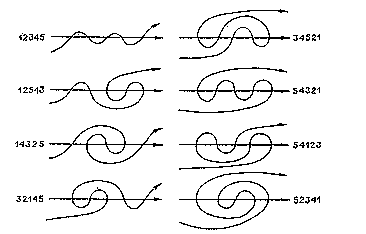Through Mazesto Mathematics

## Where else do the maze numbers occur?

At the same time (1986-88) that I was investigating the maze numbers {M(n) = number of different S.A.T. mazes with n levels} other people in different parts of the world were thinking about the same mathematical problem.

### V. I. Arnol'dAn identical counting problem was proposed by V. I. Arnol'd in Moscow; it is related to his work which appeared in the Siberian Mathematical Journal of September-October 1988. Arnol'd had defined a meander as a connected oriented non-self-intersecting curve which intersects a fixed oriented line in several points. His article contains this figure which, if tilted to the right, shows exactly the eight maze-paths of depth 6, and his M5 is the maze number M(6). Arnol'd gives the first sixteen "meandering numbers," up to M16 = M(17) = 252939.

### Vladimir A. Kazakov - Ivan Kostov

At approximately the same time, the calculation of M(n) came up in another context, this time in the computation of a matrix integral arising in quantum field theory. I learned of this work from Alexander Zvonkin, whom I thank for his correspondence. Later, he and Lando published an account of this work in their 1993 paper.

### Warren Smith

There was another simultaneous and completely independent occurrence of this problem. Warren Smith, working on a new, subexponential traveling salesman algorithm in his 1988 Princeton thesis defined jt(N) as the number of topologically distinct ways in which a Jordan curve can cross an oriented line in the plane at exactly 2N points, and computed up to jt(10) = M(20) = 8152860.

(May 13 2013) This page has been translated into Ukrainian by http://coffeehealtheffects.com.